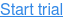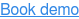# Calculate

The Calculate building block applies an arithmetic operation on two numbers. In other words, values A and B can be added, subtracted, multiplied and divided by each other, with optional rounding. See the Use Numbers and Variables lesson for examples of how this works. Fully expanded, the Calculate block shows the following properties:There are no green input or output connectors in the header, because this building block is never explicitly executed. Rather, it is used to perform a calculation on values and deliver the result to other building blocks.

The title of the block (“Calculate”) can be changed by double-clicking on it and typing in a new title.

## Value A

The first value to use in the calculation.

## Value B

The second value to use in the calculation.

## Calculation method

Select the method of calculation (addition, subtraction, multiplication or division).

## Result number

The result of the calculation.

## Round result

Specify if the result should be rounded to the specified number of decimals (digits).

Midpoint values are rounded to the nearest even number. This means that 4,45 rounded to one decimal is rounded to 4,4 and 4,35 rounded to one decimal is also rounded to 4,4.

## Round decimals

Specify the number of decimals (digits) to round the result to.

Updated April 20th 2018.